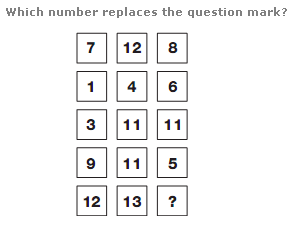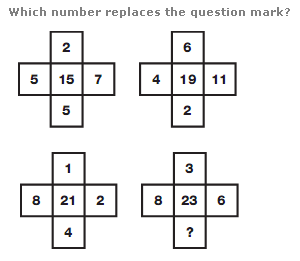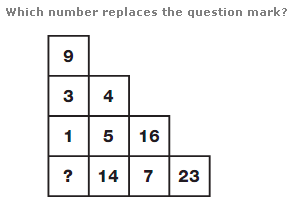# Puzzles - Number puzzles

### Exercise :: Number puzzlesAnswer : 4 Explanation : Working in rows, add the left and right hand values together and subtract 3 to give the number in the centre of each row.Answer : 4 Explanation : Add together the four outer numbers of each grid. This answer is the central number of the grid one place further clockwise.Answer : 10 Explanation : Working in columns, the sum of the numbers in each column equals 23.